# Assignments

Equations with root (radical) functions can be solved by removing the root function. If we have square roots this can be done by squaring the root function, but it takes some steps. We always have to look at the admitted values for.

1. Solve: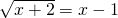Solution

2. Solve: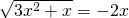Solution

3. Solve: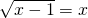Solution

4. Solve: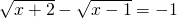Solution

5. Given the line:and the function: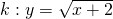For which values(s) of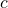doand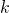have at least one point in common.

Solution

6. Solve: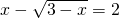Solution

7. Solve: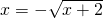Solution

8. Solve: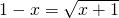Solution

9. For which value(s) ofis the line: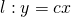tangent to the graph of: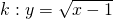Solution

10. Solve: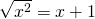Solution

0